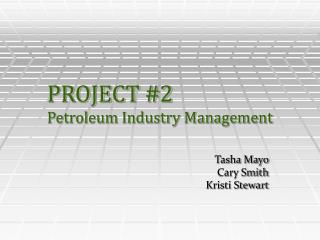# PROJECT #2 Petroleum Industry Management - PowerPoint PPT PresentationDownload PresentationPROJECT #2 Petroleum Industry Management

PROJECT #2 Petroleum Industry Management
Download Presentation## PROJECT #2 Petroleum Industry Management

- - - - - - - - - - - - - - - - - - - - - - - - - - - E N D - - - - - - - - - - - - - - - - - - - - - - - - - - -
##### Presentation Transcript

1. PROJECT #2 Petroleum Industry Management Tasha MayoCary SmithKristi Stewart

2. SYSTEM OF EQUATIONS Factory 1: 20(M) + 10 (D) + 5 (G) + 3 (P) Factory 2: 4 (M) + 14 (D) + 5 (G) + 5 (P) Factory 3: 4 (M) + 5 (D) + 12(G) + 2 (P) We need to find 3 scalars, a, b & c such that: a(F1) + b(F2) + c(F3) = Demand Current demand is: 5000 (M) + 8500 (D) + 10000 (G) **under current demand, Paraffin is not needed, so we will not consider it in our initial matrix

3. CALCULATIONS a(F1) + b(F2) + c(F3) = Demand REDUCES TO:

4. EVALUATE We must now find a, b & c as using the following equations: 5a + b + c = 1250 4b +11c = 8750 c = 675 Using back substitution, we get: 4b + 11(675) = 8750 4b + 7425 = 8750 4b = 8750 b = 331.25 5a + 331.25 + 675 = 1250 5a = 243.75 a = 48.75

5. BARREL DISBURSEMENT Since we cannot allot partial barrels, it is necessary to round up to the next whole number. The following production will meet current daily demand: a(F1) + b(F2) + c(F3) = 5000(M) + 8500(D) + 10000(G) F1: 49(20M + 10D + 5G) = 980M + 490D + 245G F2: 332(4M + 14D + 5G) = 1328M + 4648D + 1660G F3: 675(4M + 5D + 12G)= 2700M + 3375D + 8100G 5008M + 8513D +10005G

6. DEMAND DOUBLED Reduces to: Using back substitution, we find values: a = 97.5, b = 662.5, and c = 1350 These values are approximately double those we found for the original demand. 5a + b + c = 2500 4b + 11c = 17500 c = 1350

7. NEW DISTRIBUTORS REDUCES TO: 5a + b + c = 500 4b + c = 1000 c = 250 Using back substitution, we get these values: a = 12.5, b = 187.5, c = 250 If we add the demand of this new distributor to our current daily demand, we find new values for a, b and c: a = 61.25, b = 518.75, and c = 925 Notice that this is roughly equal to the number of barrels for each demand (per factory) added together.

8. SENSITIVITY ANALYSIS • - 3% change in demand for gasoline: Gives the following values: a = 53.25 (+ 9.2% change) b = 338.75 (+ 2.3% change) c = 655 (-3% change) • +3% change in demand for gasoline: Gives the following values: a = 44.25 (-9.2% change) b = 323.75 (-2.3% change) c = 705 (+ 4.4% change) These values do not fluctuate much, and production is still easily met for slight changes in demand.

9. PLANT OFF LINE • Set up augmented matrix with just F1 and F2 • Reduce • We find a system of equations that imply 0=3375, which is not possible. • Current daily demand for all products cannot be met • Suggest meeting demand for motor oil and diesel • Supply of Gasoline is the resulting amount manufactured as a result

10. OTHER PROBLEMS • 4TH Plant acquisition? • Production equal to F3 • We find values: a = 48.75, b = 331.25, and c=d=337.5 • The values for c and d are half of the original value for c we found when only 3 plants were running. • Allows for partial shutdown, repairs. • Paraffin supply: • a(F1) + b(F2) + c(F3) = 49(3p) + 332(5p) + 675(2p) • Total production of 3157 gallons of paraffin per day

11. SELLING FACTORY #1 • Cannot meet current daily demand for motor oil without excess production of diesel and gasoline • Suggest meeting demand for diesel and gasoline, and selling whatever amount of motor oil is produced with the number of barrels supplied: • Set up augmented matrix with value for motor oil set to variable • Solve for diesel and gasoline. • Values found: a = 364, b = 682, (variable for M = 4184) • These numbers of barrels will meet supply of diesel and gasoline without excess production, yet will only produce 4184 gal of Motor Oil. • Note that utilizing F4 would not help in the production of Motor oil without producing excess diesel and gasoline.

12. CONCLUSION • Each plant has a unique # of barrels needed to produce current daily demand. • Modest changes in demand can be met with all 3 plants in operation. • Changes in production capability can drastically alter the company’s ability to meet demand. • Finding buyers for waste products (such as paraffin) may be beneficial.